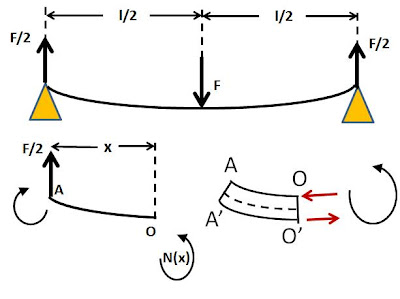## Saturday, April 11, 2009

### Irodov Problem 1.302In order to keep the beam in equilibrium, the two ends must provide a total reaction force of F in the upward direction. The reaction forces offered by each of the two ends must exactly be the same since otherwise a torque will be induced in the beam causing it to rotate about its center. Hence, each of the ends offer a force of F/2 in the upward direction.

Now let us consider the torque acting on a section AO of the beam, such that O is at coordinate x from the left end. The reaction force F/2 causes a torque in the clockwise direction of magnitude Fx/2. This must be compensated by the elastic moment in the beam N(x). In other words N(x) must act in the counter-clockwise direction and must be equal to Fx/2 in magnitude. The elastic moment in the beam can be in the counter-clockwise direction only if the lower surface of the beam AO is stretched more than the lower surface A'O' as shown in the figure. This means that the elastic curve of the beam must be a convex function i.e.. We also know that the elastic cruve follows the equation,(as given in Irodov problem 1.301 or the link).

Hence we have,